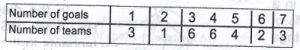# Mathematics 2018 Past Questions | WAEC

Study the following Mathematics past questions and answers for JAMBWAEC  NECO and Post-JAMB. Get prepared with official past questions and answers for upcoming examinations.

11.  $$\frac{2 – 18m^2}{1 + 3m}$$

• A. $$2 (1 + 3m)$$
• B. $$2 (1 + 3m^2)$$
• C. $$2(1 – 3m)$$
• D. $$2(1 – 3m^2)$$

12. A curve is such that when y = 0, x = -2 or x = 3. Find the equation of the curve.

• A. y =  ${\mathrm{x²}}^{}-5x-6$
• B. y =  ${\mathrm{x²}}^{}+5x-6$
• C. y =  ${\mathrm{x²}}^{}+x-6$
• D. y =  ${\mathrm{x²}}^{}-x-6$

13.  The volume of a cylindrical tank, 10m high is 385 m2. Find the diameter of the tank. [Take π=22/7]

A. 14m
B. 10m
C. 7m
C. 5m

14.  The surface area of a sphere is 792/7cm2. Find, correct to the nearest whole number, its volume. [Take π=22/7]

A. 113cm3
B. 131cm3
C. 311cm3
D. 414cm3

15. The angles of a polygon are x, 2x, 2x, (x + $$30^o$$), (x + $$20^o$$) and (x – $$10^o$$). Find the value of x

• A. $$45^o$$
• B. $$95^o$$
• C. $$84^o$$
• D. $$85^o$$

16. If M and N are the points (-3, 8) and (5, -7) respectively, find |MN|

• A. 8 units
• B. 11 units
• C. 15 units
• D. 17 units

17.  The equation of the line through the points (4,2) and (-8, -2) is 3y = px + q, where p and q are constants. Find the value of p.

• A. 1
• B. 2
• C. 3
• D. 9

18. The mean of 1, 3, 5, 7 and x is 4. Find the value of x

• A. 2
• B. 4
• C. 6
• D. 8

19.The table shows the distribution of goals scored by 25 teams in a football competition. Calculate the probability that a team selected at randon scored either 4 or 7 goals.

• A. 9/25
• B.  1/5
• C.  6/25
• D.  $\frac{2}{}$/5

20.The table shows the distribution of goals scored by 25 teams in a football competition. Calculate the probability that a team selected at random scored at most 3 goals.

• A. 3/25
• B. 1/5
• C. 6/25
• D. 2/5
•
Scroll to Top